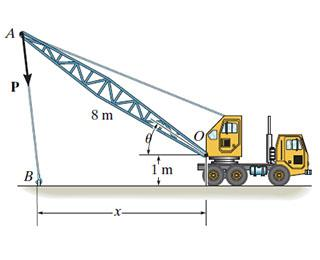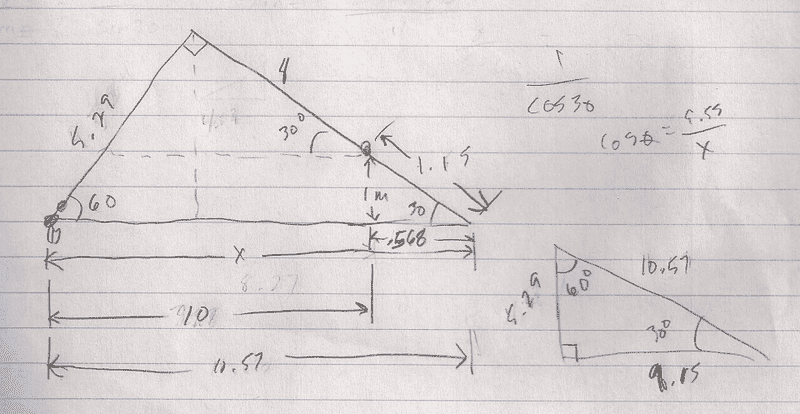# Moment about a point of a crane

## Homework StatementThe towline exerts a force of P = 5.8 kN at the end of the 8-m-long crane boom.

If θ = 30∘ determine the placement x of the hook at B so that this force creates a maximum moment about point O.

## The Attempt at a Solution

M(max)= 8(5800) = 4.64kN*m

My initial thought was to find the components of P but there is not enough info for that. I've seen some other work equating the x & y distances to M(max) but without another angle I'm not sure where to go.

lewando
Homework Helper
Gold Member
What must the angle between the boom and towline be for maximum moment (torque) about O?

•PhanthomJay
90. So the angle between P and the x axis is 60?

5.8sin60 - 5.8cos60(x) = 4.64kN*m

lewando
Homework Helper
Gold Member
Yes. It becomes a geometry problem now. Not sure where you are going with your equation. When you solve for 'x' does it make sense?

No, I guess not. The magnitude of the vertical force component = 4.64sin(60)= 4.02kN*m. Not sure from there. Obviously the horizontal force component is 4.64cos(60)= 2.32kN*m but I'm not sure what to do with that info. I know the vertical component gets multiplied by the horizontal distance and the horizontal by the vertical distance.

lewando
Homework Helper
Gold Member
You are trying to find the distance 'x'. At this point, the distance 'x' is the same if there is 5.8 kN on the towline or 1 N on the towline. Look at the triangle(s) formed.

Last edited:
lewando
Homework Helper
Gold Member
Looks good. But that is almost a triangle. Add a mini triangle to the right-hand side by extending AO down to the base and from O straight down to the base. You have enough information to determine the lengths of the sides of the mini and therefore the big one.

So, the new boom length ( with the samll triangle) is 9.15m. The leg opposite the 30 degree angle is 5.29 and the hypotenuse is 10.57 (x value). Subtract .568 (base of small traingle) from 10.57 and x=10.

lewando
Homework Helper
Gold Member
Can you repost your new picture. Something does not seem right.lewando
Homework Helper
Gold Member
The lengths of the two sides of the small triangle are switched.

lewando
Homework Helper
Gold Member
Not switched--I mean not right.

r=1/cos(30)= 1.15
x=sqrt(1.15^2-1)= .568

The base of the small triangle is the hypotenuse of the large triangle.

lewando
Homework Helper
Gold Member
If r is the hypotenuse of the small triangle then sin(30) = 1/r. "Opposite/hypotenuse".

So side adjacent 30 degree angle is 10m, side opposite is 5 and hypotenuse is 11.18. Adjacent side on small triangle is 1.732. X=1.732-11.18=9.448m. I'm assuming this is not the x I need. Looking at the drawing, that length (9.448) seems counter intuitive.

lewando
Homework Helper
Gold Member
True: big triangle--side adjacent to 30-degree angle is 10 m.
False: big triangle--side opposite is 5 m.
False: big triangle--hypotenuse is 11.18.

Recall the 1, 2, sqrt(3) proportion of a 30/60/90 triangle.

By the way, Robb--mental overload...been there many times. Take a step back, walk away slowly, re-engage!

Last edited:
Ok. Base = 10; Side opposite 30 degree angle=5.77; hypotenuse(x)=11.54

lewando
Homework Helper
Gold Member
All looks correct except for relating 'x' with the hypotenuse. 'x' is less than the hypotenuse of the big triangle.